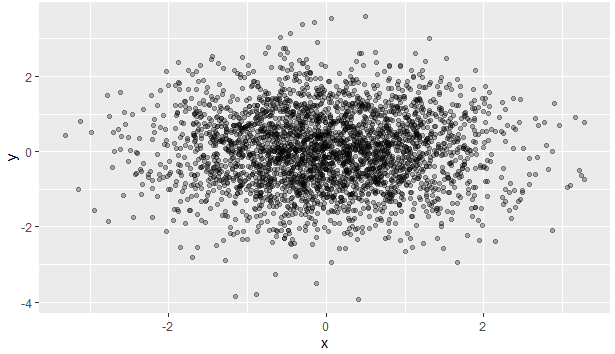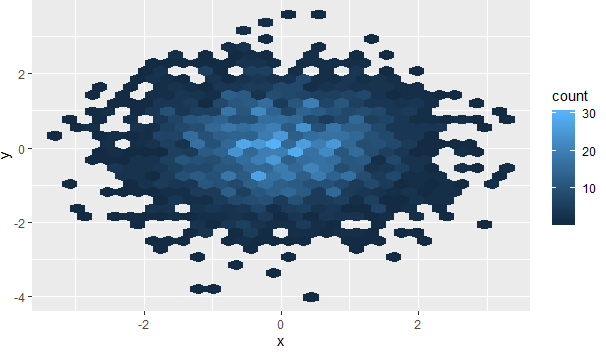4 views

I am trying to plot two variables where N=700K. The problem is that there is too much overlap so that the plot becomes mostly a solid block of black. Is there any way of having a grayscale "cloud" where the darkness of the plot is a function of the number of points in a region? In other words, instead of showing individual points, I want the plot to be a "cloud", with the more the number of points in a region, the darker that region.

by

To avoid overlapping of points in a scatterplot, you can use the alpha function that is used to modify color transparency.

For example:

df <- data.frame(x = rnorm(3000),y=rnorm(3000))

ggplot(df,aes(x=x,y=y)) + geom_point(alpha = 0.3)

Output:You can also use hexagonal binning with the help of the hexbin package as follows:

ggplot(df,aes(x=x,y=y)) + stat_binhex()

Output: